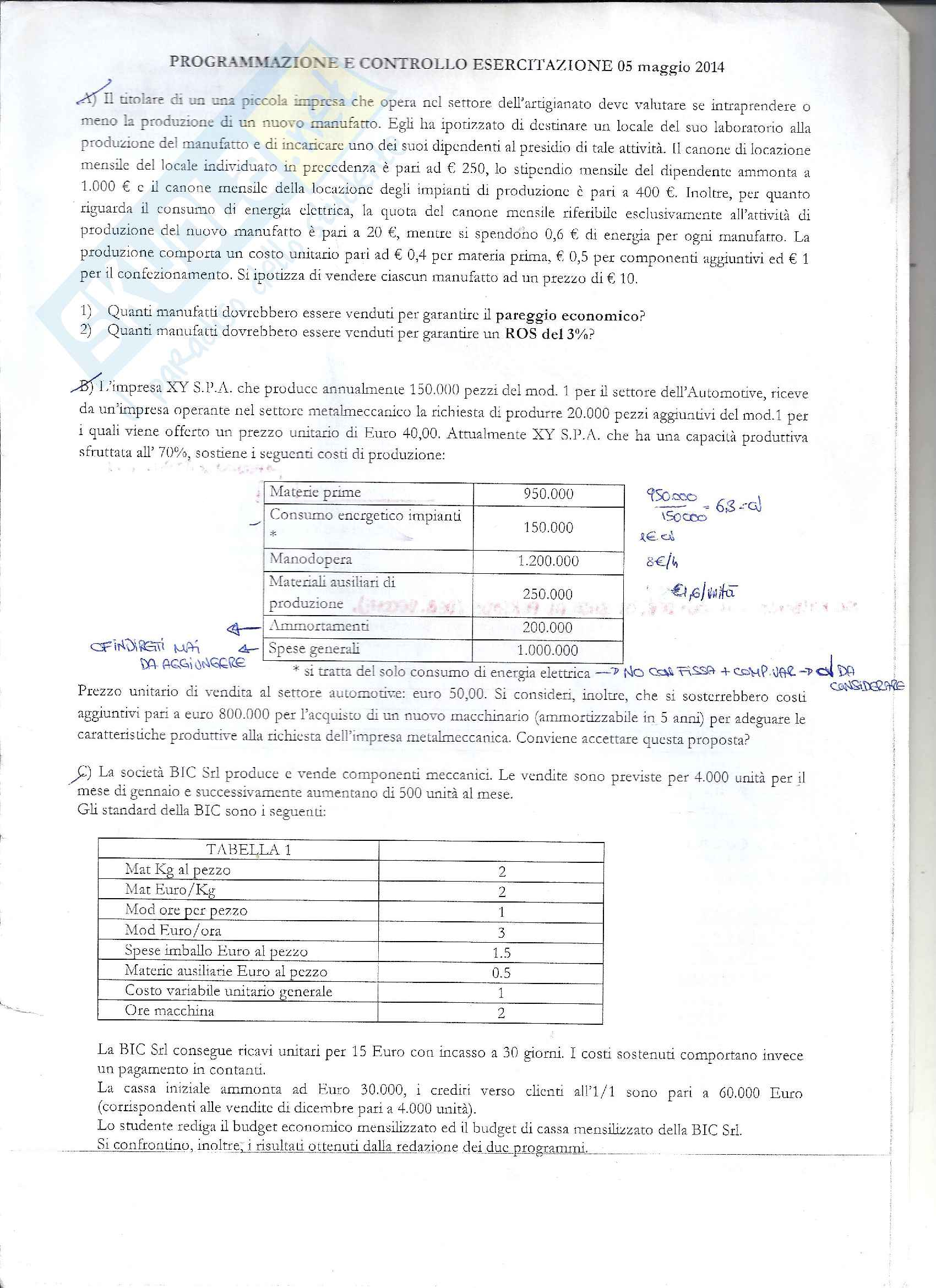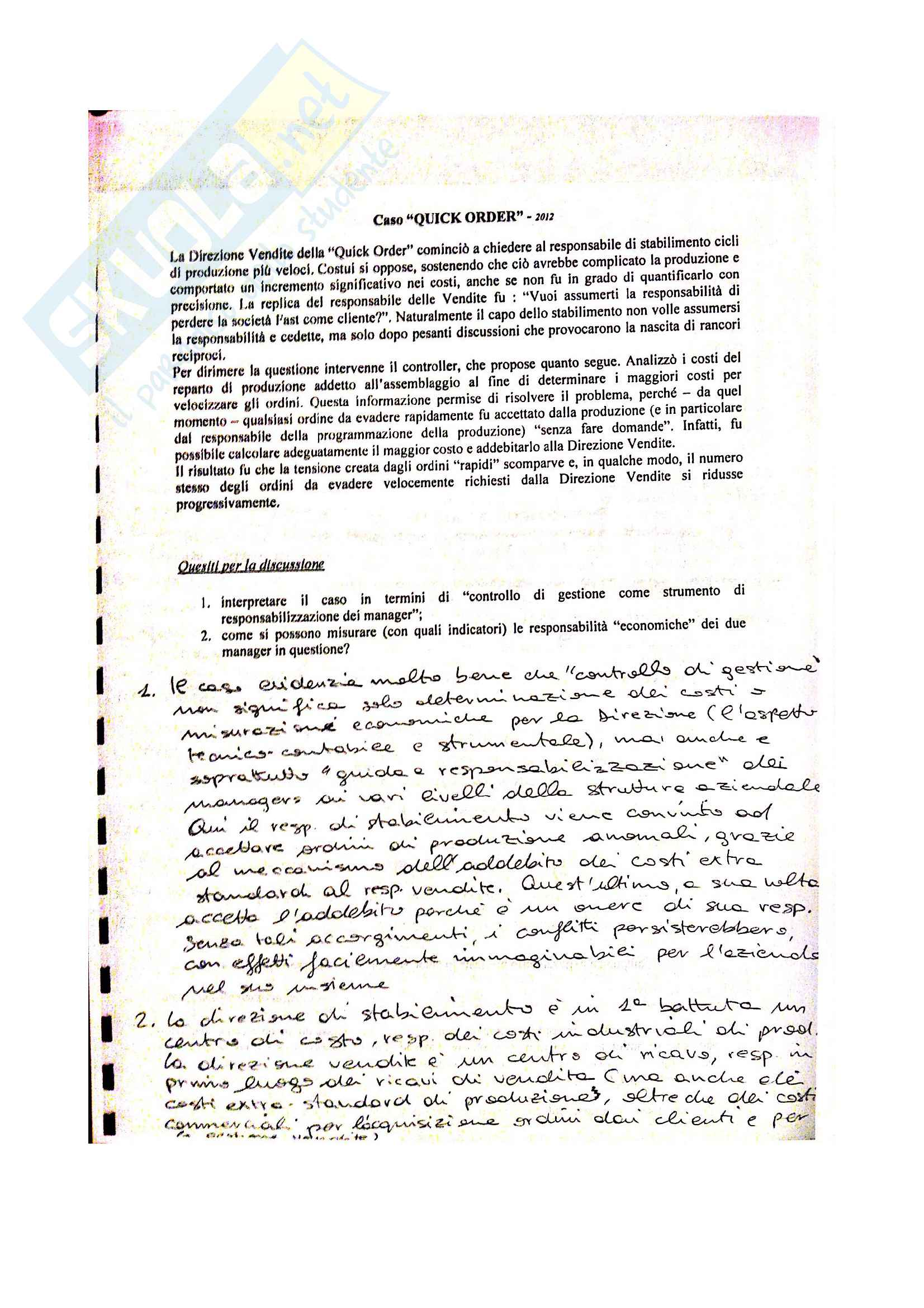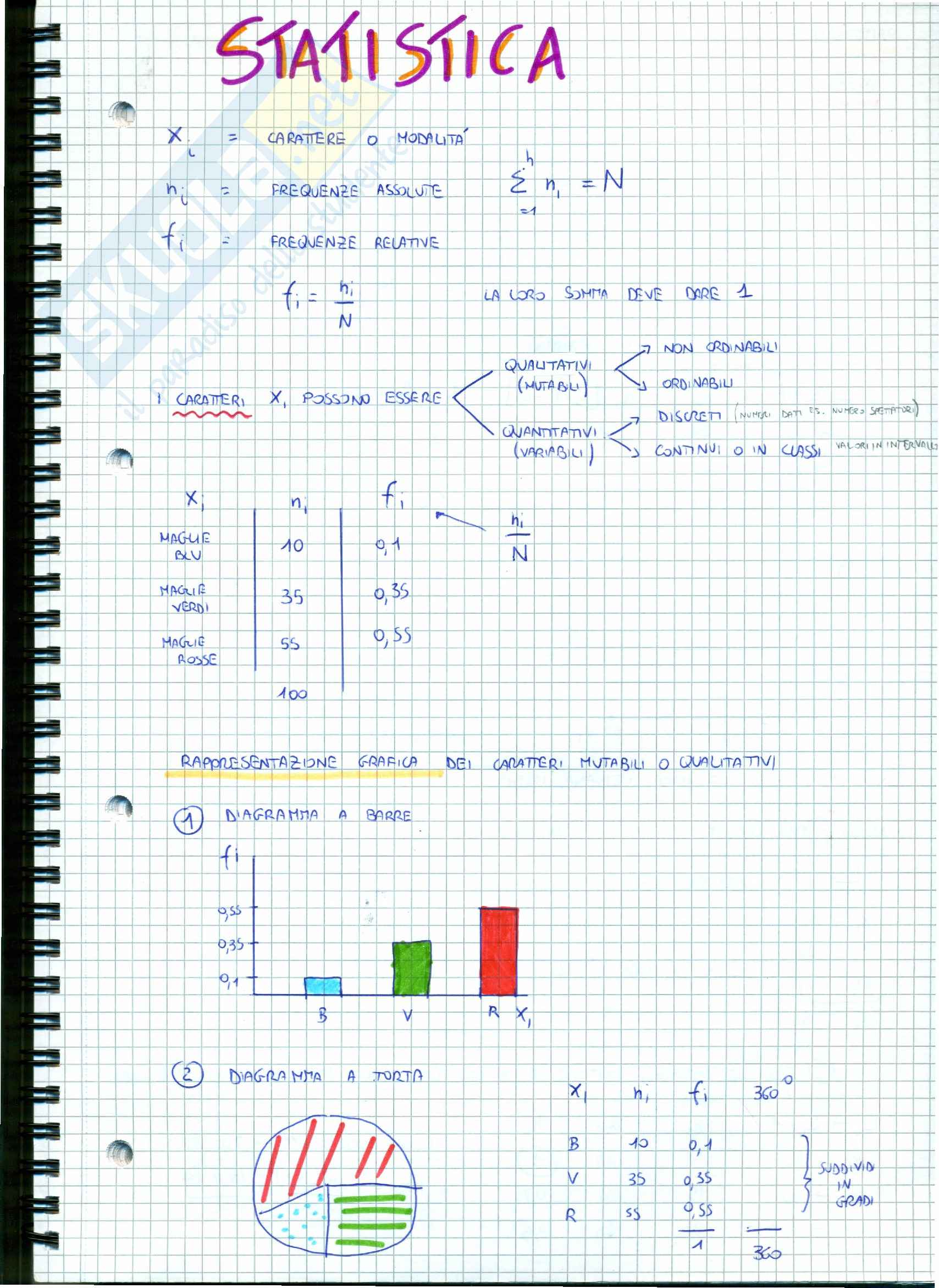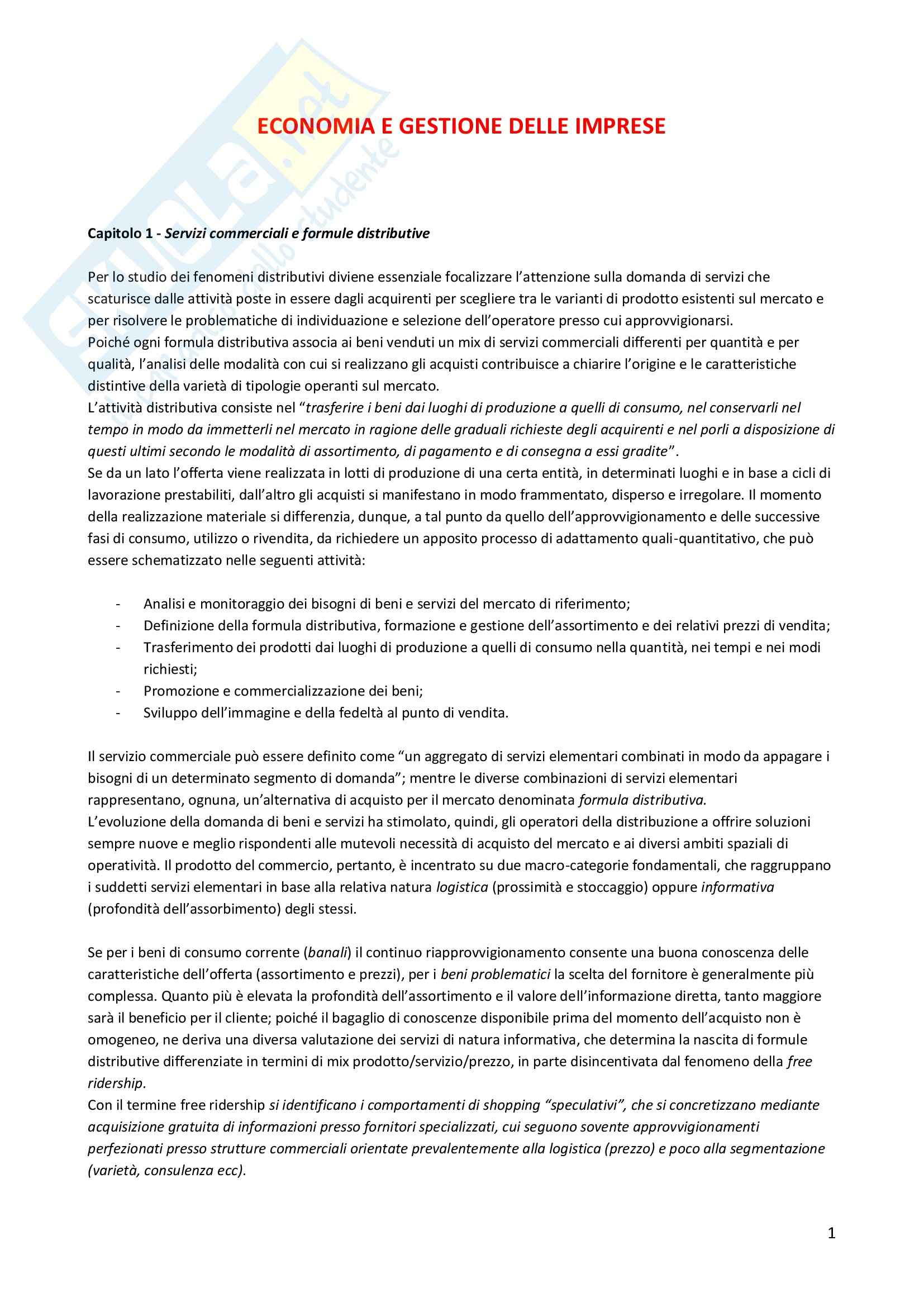Che materia stai cercando?

# Riassunto esame Microeconomics, prof. Vannoni, libro consigliato Microeconomics, PindyckRiassunto in inglese per l'esame di Microeconomics, basato su appunti personali e studio autonomo del testo Microeconomics di Pindyck consigliato dal docente Davide Vannoni, facoltà di Economia all'università degli studi di Torino, corso di laurea in Business Management.

Esame di Microeconomics docente Prof. D. Vannoni

Anteprima

### ESTRATTO DOCUMENTO

If income increase it shifts to the right, is price of a single good increase or decrease it just

rotates. Its slope is –(Pf on Pc)

3. Consumer choice

In the end, the consumer buys what , among its possible preferred baskets, is affordable within

its budget. So, the optimal choice is the point where the indifference curve with the highest

UTILITY, level of satisfaction, intersects the budget line. In other words it is where

MRS= Pf/PC= MUf/MUc

## OPTIMAL CHOICE FOR A CONSUMER

MARGINAL UTILITY, MU, is the additional satisfaction obtained from consuming one

Individual and market demand

Demand function> prices vary> optimal choices vary> we have different optimal choices>the curve

that joins the various optimal choices is the PRICE CONSUMTION CURVE.

Demand function> income varies> optimal choices vary> we have different optimal choices> the

curve that joins them is the INCOME CONSUMPTION CURVE, also known as the ENGEL

CURVE. This curve is an upward line for normal goods, but a curve that is first upward and then

downward in case of inferior goods, those good like hamburger that when income increase lose

market. In fact as soon as income increase I consume more of hamburger and steak, but then I

prefer consuming more of steak that is more expensive and good and I reduce the consumption of

hamburger.

For example, if price of food decreases, then I consume more food and less clothes, this is the

## SUBSTITUTION EFFECT.

Then>

• if the good is normal, the income effect is positive, but lower than substitution

• if the good is inferior, the income effect is negative, but lower than substitution

• if the good is a Giffen good, the income effect is negative and greater than the substitution

one.

Production

In producing, a firm must consider how many inputs to use. A production function says it. This

inputs are labor and capital. In the short run at least one input must be fixed, in the long run a firm

can change both inputs.

• Production in the short run> one variable input> labor

Here, the firm has a fixed capital so the only option It has to increase its output its increasing

labor.

How many units of labor will a firm decide to use as an input?

It depends if benefits of hiring more people are higher than costs.

We can decide it analyzing the situation on an incremental basis, how output increases when an

additional unit of labor is added, or on an average one, how much output each worker produces.

2

Amount of labor Amount of Total output Average product Marginal product

L capital q Q/L DeltaQ/DeltaL

## K

Increases Fixed Increases and Increases and Increases and

then decreases then decreases then decreases

The product curve that represents the relation between output and labor , at first goes up but

after a certain amount of labor added it goes down.

The average product of labor curve, is the slope of the line that goes from the origin of the

product curve to one of its points.

The marginal product of labor curve, is the slope of a a line that is tangent to a point on the

product curve.

They both increase and then decrease. So we have diminishing marginal returns.

When the marginal product is over the average product, the average product is increasing.

When the marginal product is under the average product, the average product is decreasing.

The marginal product increases faster than the average one.

• Production in the long run> two variable inputs> labor and capital

Here we can change both inputs. Let’s see how many combinations we have, keeping output

fixed. The various possible combinations of both inputs are all points of an ISOQUANT. Each

isoquant corresponds to a different level of production. All the possible combinations on the

same isoquant give rise to the same quantity of output. The isoquant is quite similar to the

indifference curve, it is downward sloping because of the diminising marginal returns.

The slope of the isoquant is the MARGINAL RATE OF TECHNICAL SUBSTITUTION,

MRTS. Since the firm remains on the same isoquant, when it decides to increase labor, it must

decrease capital by the same amount to keep on having the same output. It is a positive quantity

like MRS:

MRTS= -(deltaK/deltaL).

This rate is diminishing because the productivity of both inputs is limited:

## INDIFFERENCE CURVE ISOQUANT

Slope: MRS=- deltaC/deltaF Slope: MRTS= - deltaK/deltaL

Diminishing marginal returns Diminishing marginal returns

## MRS=MUF/MUC MRTS= MPL/MPK

By now we have just considered the possibility of substituting inputs to get the same output

level.

Now we consider also the possibility of increasing output by increasing inputs.

What happens to the production level?

1. input doubles> output doubles, constant returns to scale, sum of the coefficients of the

function is = 1, isoquants are equidistant

2. input doubles> output more than doubles, increasing returns to scale, sum >1, isoqants are

close together

3. input doubles> output less than doubles, decreasing returns to scale, sum< 1, isoquants are

far apart 3

The cost of production

• cost in the short run

As we have diminishing marginal returns in the production, output decreases when adding labor

after a certain level, so this means I need more labor to produce an amount of output, so I have

more costs, so the marginal cost( cost of producing one unit more) is increasing.

Marginal product is decreasing(diminishing marginal returns) > marginal cost is increasing

Fixed costs decrease with output.

When marginal cost is under the average cost, the average cost decreases.

When marginal cost is over the average cost, the average cost increases.

Average cost curve is U shaped because of diminishing marginal returns.

• Cost in the long run

Here we can change both inputs, so we can choose those inputs with the lowest costs to have the

same output.

1. The firm wants the output to be fixed.

We use ISOCOST LINES, whose function is the total cost one and whose slope is

deltaK/deltaL. They are similar to the budget line.

Where the isoquant intersects the lowest isocost that is the Optimal choice in terms of costs.

MRTS=MPL/MPK=w/r

## OPTIMAL CHOICE OF PRODUCTION IN TERMS OF COSTS, MINIMIZING THEM

Average cost curve in the long run is U shaped because of the returns to scale:

• Constant returns to scale> same prices> same costs

• Increasing returns to scale> lower prices> lower costs

• Decreasing returns to scale> higher prices> higher costs

Where marginal cost is under average cost, average cost is decreasing

Where marginal cost is over the average cost, the average cost is increasing.

2. Output level changes > isoquants change> we have different optimal choices>expansion path

joins all the points of choices.

In the short run, because og the inflexibility of capital, the expansion path is a horizontal line

In the long run, the expansion path is a line from the origin.

Conclusions on both chapter 6 and 7:

If output doubles and costs less than double> economies of scale

If output doubles and costs more than double> diseconomies of scale

Economies and diseconomies of scale depend on the elasticity of cost on quantity>

Ec= (deltaC/deltaQ)/ (C/Q)= MC/AC

• If Ec=1> , MC=AC, nothing

• IF Ec>1, MC>AC, diseconomies of scale

• If Ec< 1, MC< AC, economies of scale

Increasing returns to scale: output more than doubles when the inputs double.

Economies of scale: output double but costs less than double 4

Profit maximization and competitive supply

Given what the demand is and what the costs are, the firm should decide how much to produce, that

is the supply function.

In perfect competition:

1. homogenous goods, products are perfectly substitutable

2. price taking, each firm takes the price as given

3. free entry and exit.

Introduction:

Marginal revenue is the slope of the revenue curve and marginal cost is the slope of the cost curve.

At the beginning when we have zero output costs are positive because we have fixed costs which do

not depend on the ouput, revenues are zero of course so the profit is negative.

Then when output increases, revenues increase and costs increase but less than revenues so profit is

finally positive until it reaches a point where it is maximum. The output level chosen is where

MC=MR. Any output lower than this would lead to MR> MC, any higher output would lead to

## MC>MR.

This point is exactly where MC=MR=P

## PROFIT MAXIMIZATION

Marginal costs and revenues are equal to price because the firm in the market is price taker so the

demand curve for a single firm is horizontal, whilst the demand curve for the industry is downward

sloping.

• Choosing an output that maximizes profit in the short run

Here we have fixed capital.

The profit is thus maximized at MC=MR and it is equal to the area of the rectangle between

ATC, MC and MR.

If fixed costs are high, it might happen that ATC is above MR=P, so there would be a

subsequent loss and the firm should better go out of the market. But, if these fixed costs are

sunk costs, then the firm should stay in the market and use as a profit the one formed by MR,

AVC and MC.

In the short run, the supply curve is then a part of the MC where MC is above AC=P.

Perfectly inelastic supply > a greater output can be achieved only if new plants are built.

Perfectly elastic supply> marginal cost is constant

The producer surplus= revenues – variable costs

Profit= revenues – variable costs- fixed costs

• Choosing output in the long run

Here, the firm can change its inputs and also decide to rearrange its plant size.

The profit is maximized where MC= P

## PROFIT MAXIMIZATION IN THE LONG RUN

If MC= ATC then profit = 0, ZERO ECONOMIC PROFIT.

In the long run the equilibrium occurs where:

1. all firms in the industry are maximizing their profit 5

PAGINE

9

PESO

86.50 KB

AUTORE

PUBBLICATO

+1 anno fa

DETTAGLI
Corso di laurea: Corso di laurea in economia aziendale
SSD:
Università: Torino - Unito
A.A.: 2013-2014

I contenuti di questa pagina costituiscono rielaborazioni personali del Publisher Marie Therese di informazioni apprese con la frequenza delle lezioni di Microeconomics e studio autonomo di eventuali libri di riferimento in preparazione dell'esame finale o della tesi. Non devono intendersi come materiale ufficiale dell'università Torino - Unito o del prof Vannoni Davide.

Acquista con carta o conto PayPal

Scarica il file tutte le volte che vuoi

Paga con un conto PayPal per usufruire della garanzia Soddisfatto o rimborsato

RecensioniTi è piaciuto questo appunto? Valutalo!

## Altri appunti di Corso di laurea in economia aziendaleEsercitazione

### Appelli svolti controllo di gestioneEsercitazione

### Temi d'esame di organizzazione aziendaleAppunto

### Matematica e statistica per l'economia - appuntiAppunto